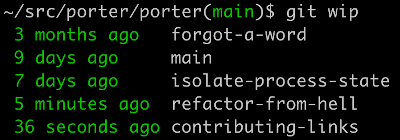Comment on page

# Data Science Tools

## Python

Async io
1. 1.
Intro
2. 2.
complete
Clean code:

### Virtual Environments

#### PYENV

1. 5.
pyenv virtualenv

## JUPYTER

• (Debugging in Jupyter, how?) - put a one liner before the code and query the variables inside a function.
• Jupyter notebooks as a module
1. 1.
Nbdev, on fast.ai
2. 2.
jupytext
1. 1.
2. 2.
\$ python -m venv projectname
3. 3.
\$ source projectname/bin/activate
4. 4.
(venv) \$ pip install ipykernel
5. 5.
(venv) \$ ipython kernel install --user --name=projectname
6. 6.
Run jupyter notebook * (not entirely sure how this works out when you have multiple notebook processes, can we just reuse the same server?)
7. 7.
Connect to the new server at port 8889
8. 8.
(how does reshape work?) - a shape of (2,4,6) is like a tree of 2->4 and each one has more leaves 4->6.
As far as i can tell, reshape effectively flattens the tree and divide it again to a new tree, but the total amount of inputs needs to stay the same. 2*4*6 = 4*2*3*2 for example
code: ```import numpy rng = numpy.random.RandomState(234) a = rng.randn(2,3,10) print(a.shape) print(a) b = numpy.reshape(a, (3,5,-1)) print(b.shape) print (b)```
How to add extensions to jupyter: extensions

## SCIPY

1. 1.
2. 2.
Minima / maxima finding it in a 1d numpy array

## NUMPY

Using numpy efficiently - explaining why vectors work faster. Fast vector calculation, a benchmark between list, map, vectorize. Vectorize wins. The idea is to use vectorize and a function that does something that may involve if conditions on a vector, and do it as fast as possible.

## PANDAS

1. 1.
Great introductory tutorial about using pandas, loading, loading from zip, seeing the table’s features, accessing rows & columns, boolean operations, calculating on a whole row\column with a simple function and on two columns even, dealing with time\date parsing.
2. 6.
3. 8.
Accessing dataframe rows, columns and cells- by name, by index, by python methods.
4. 11.
1. 1.
Create a new column based on a (boolean or not) column and calculation:
2. 2.
Using python (map)
3. 3.
Using numpy
4. 4.
using a function (not as pretty)
5. 12.
Given a DataFrame, the shift() function can be used to create copies of columns that are pushed forward (rows of NaN values added to the front) or pulled back (rows of NaN values added to the end).
1. 1.
df['t'] = [x for x in range(10)]
2. 2.
df['t-1'] = df['t'].shift(1)
3. 3.
df['t-1'] = df['t'].shift(-1)
6. 14.
Dataframe Validation In Python - A Practical Introduction - Yotam Perkal - PyCon Israel 2018
7. 15.
In this talk, I will present the problem and give a practical overview (accompanied by Jupyter Notebook code examples) of three libraries that aim to address it: Voluptuous - Which uses Schema definitions in order to validate data [https://github.com/alecthomas/voluptuous] Engarde - A lightweight way to explicitly state your assumptions about the data and check that they're actually true [https://github.com/TomAugspurger/engarde] * TDDA - Test Driven Data Analysis [ https://github.com/tdda/tdda]. By the end of this talk, you will understand the Importance of data validation and get a sense of how to integrate data validation principles as part of the ML pipeline.
8. 16.
Stop using itterows, use apply.

### Exploratory Data Analysis (EDA)

1. 3.
Sweetviz - "Sweetviz is an open-source Python library that generates beautiful, high-density visualizations to kickstart EDA (Exploratory Data Analysis) with just two lines of code. Output is a fully self-contained HTML application.
The system is built around quickly visualizing target values and comparing datasets. Its goal is to help quick analysis of target characteristics, training vs testing data, and other such data characterization tasks."by Sweetviz

### TIMESERIES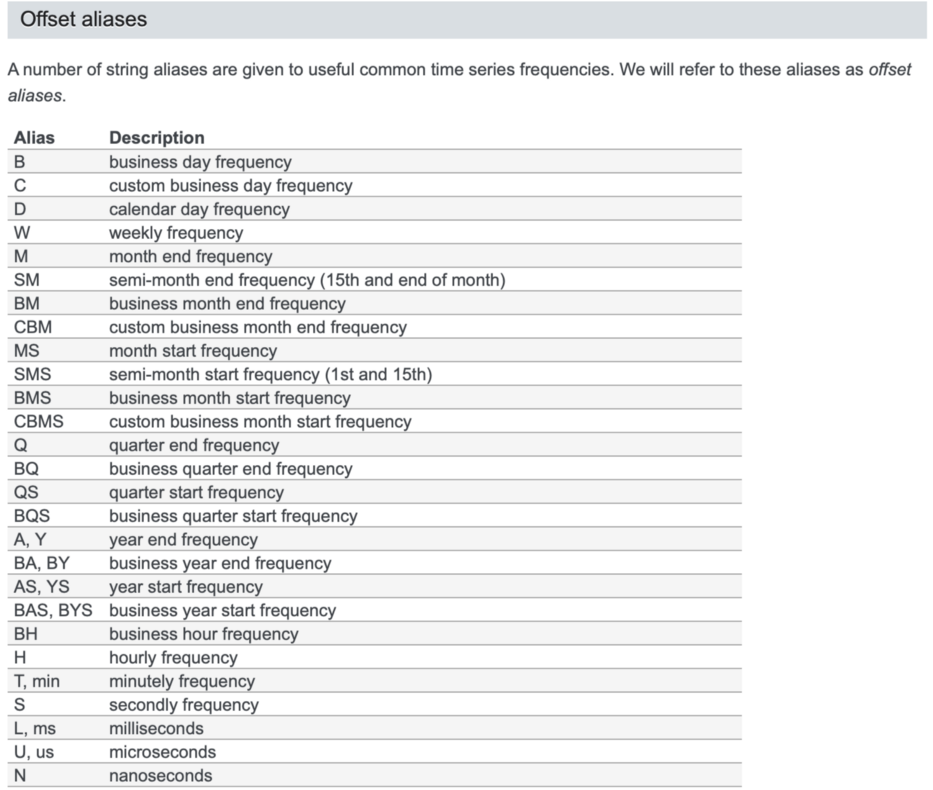1. 3.
SCI-KIT LEARN
2. 4.
Pipeline to json 1, 2
3. 5.
cuML - Multi gpu, multi node-gpu alternative for SKLEARN algorithms
4. 6.
5. 7.
Awesome code examples about using svm\knn\naive\log regression in sklearn in python, i.e., “fitting a model onto the data”
Also Insanely fast, see here.
1. 1.
Functional api for sk learn, using pipelines. thank you sk-lego.
2. 2.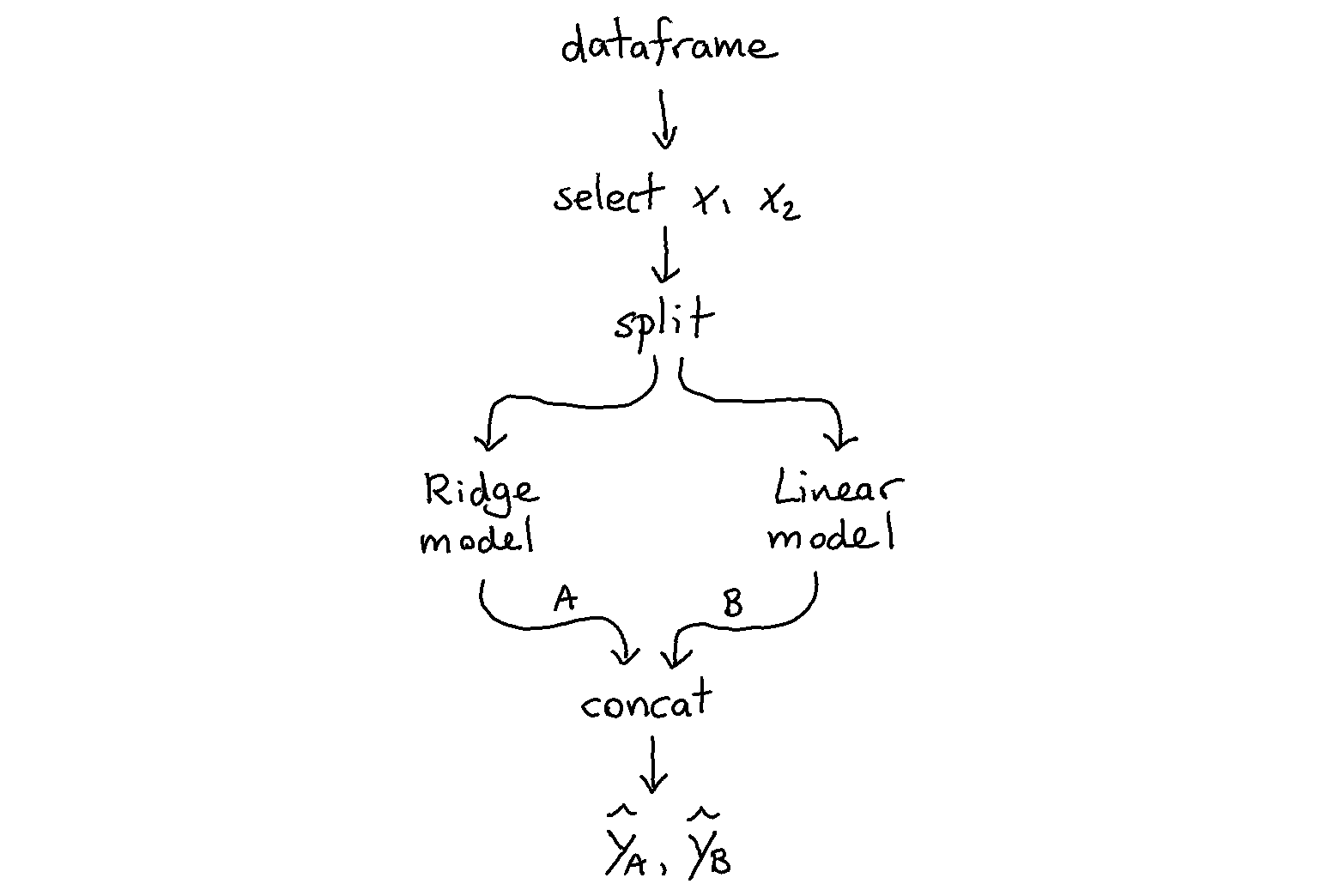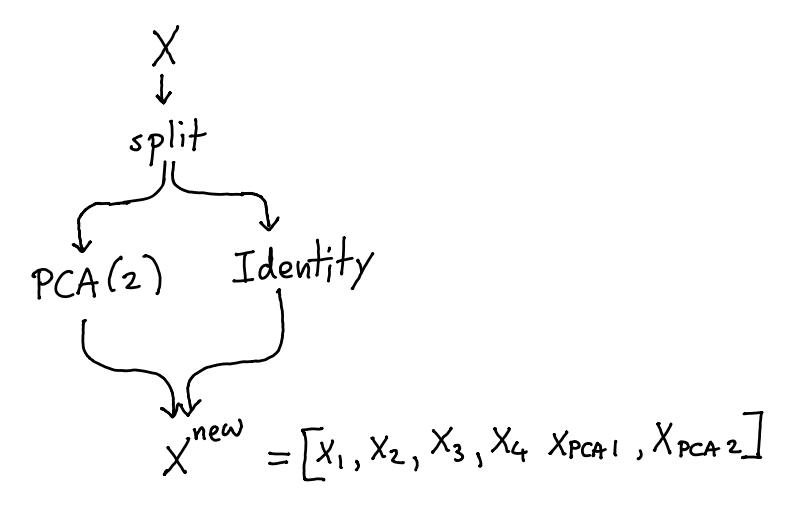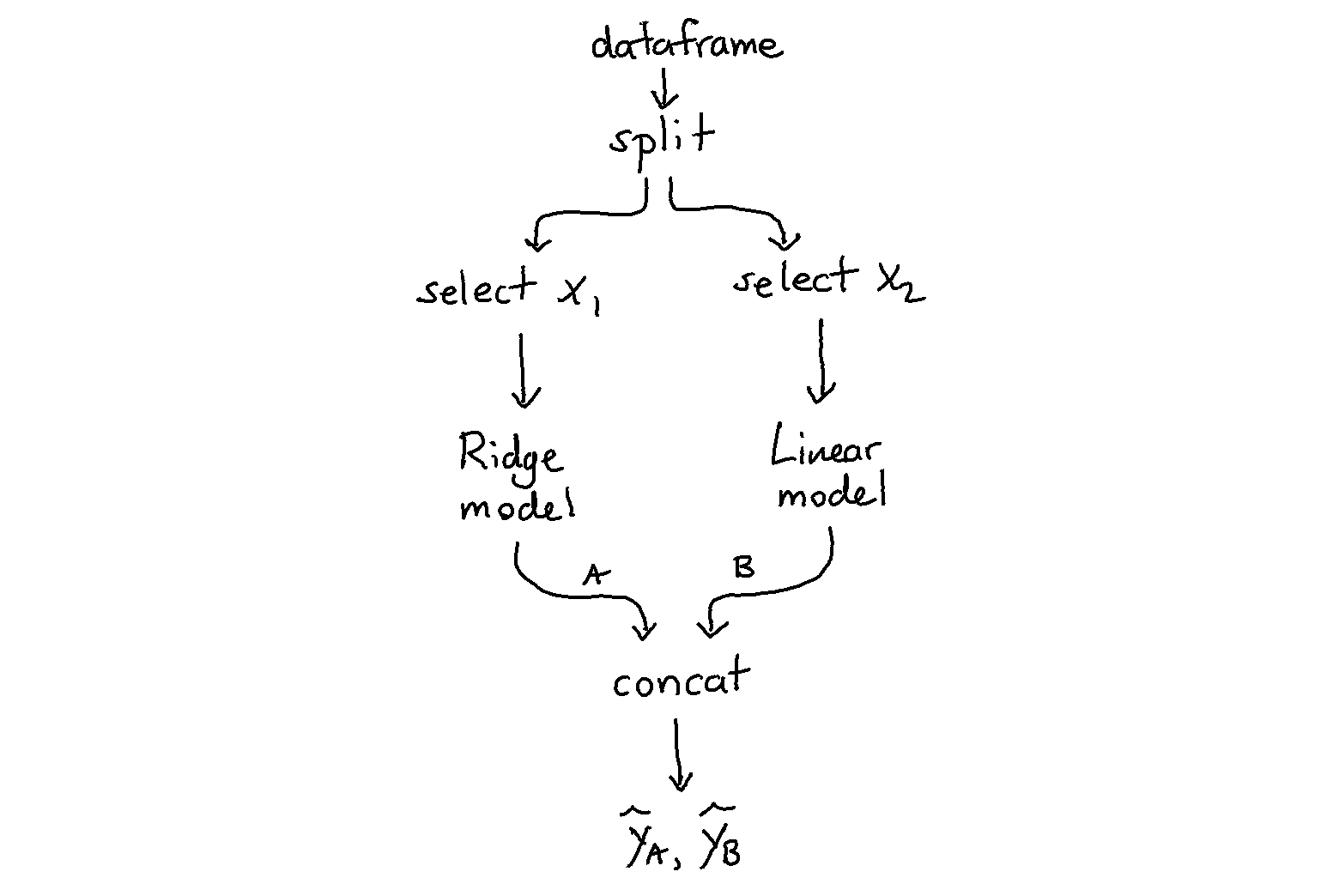Images by SK-Lego

## FAST.AI

1. 1.
Medium on all fast.ai courses, 14 posts

## PYCARET

1. What is? by vidhaya - PyCaret is an open-source, machine learning library in Python that helps you from data preparation to model deployment. It is easy to use and you can do almost every data science project task with just one line of code.

## NVIDIA TF CUDA CUDNN

• Failed to initialize NVML: Driver/library version mismatch

## GIT / Bitbucket

1. 2.
2. 5.
3. 7.
Git wip (great)